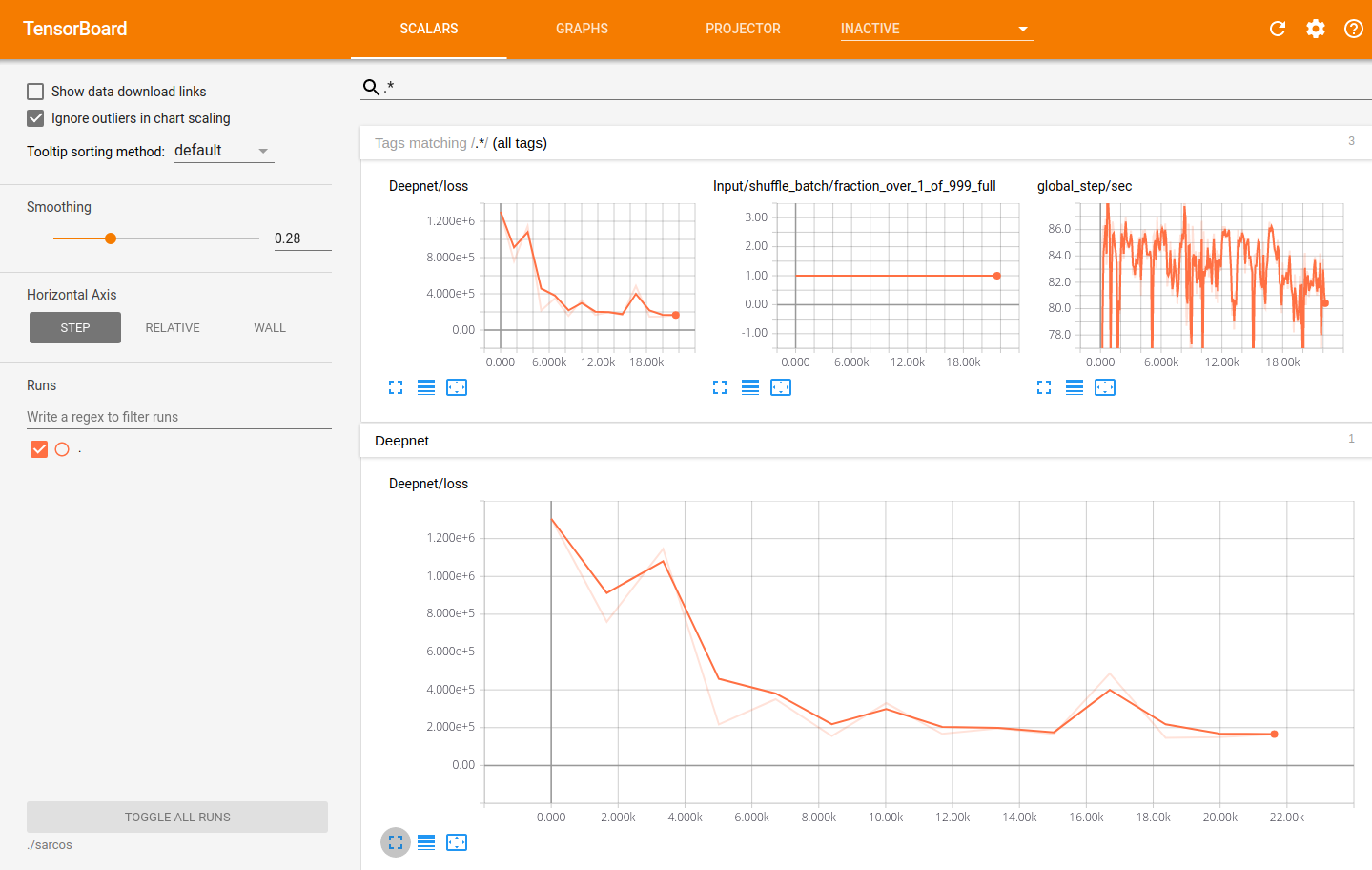# Demos¶

We have included some demonstration scripts with Aboleth to help you get familiar with some of the possible model architectures that can be build with Aboleth. We also demonstrate in these scripts a few methods for actually training models using TensorFlow, and how to get up and running with TensorBoard, etc.

## Regression¶

This is a simple demo that draws a random, non linear function from a Gaussian process with a specified kernel and length scale. We then use Aboleth (in Gaussian process approximation mode) to try to learn this function given only a few noisy observations of it. This script also demonstrates how we can divide the data into mini-batches using utilities in the tf.train module, and how we can use tf.train.MonitoredTrainingSession to log the learning progress.

This demo can be used to generate figures like the following:You can find the full script here: regression.py.

## SARCOS¶

Here we use Aboleth, again in Gaussian process regression mode, to fit the venerable SARCOS robot arm inverse kinematics dataset. The aim is to learn the inverse kinematics from 44484 observations of joint positions, velocities and accelerations to joint torques.

This problem is too large for a regular Gaussian process, and so is a good demonstration of why the approximation in Aboleth is useful (see Approximate Gaussian Processes). It also demonstrates how we learn automatic relevance determination (ARD, or anisotropic) kernels.

We have also demonstrated how you can use TensorBoard with the models you construct in Aboleth, so you can visually monitor the progress of learning. This has the nice side-effect of also enabling model check point saving, so you can actually resume learning this model if you run the script again!!Using TensorBoard to visualise the learning progress of the Aboleth model fitting the SARCOS dataset.

This demo will make a sarcos folder in the directory you run the demo from. This contains all of the model checkpoints, and to visualise these with TensorBoard, run the following:

\$ tensorboard --logdir=./sarcos


The full script is here: sarcos.py.

## Multiple Input Data¶

This demo takes inspiration from TensorFlow’s Wide & Deep tutorial in that it treats continuous data separately from categorical data, though we combine both input types into a “deep” network. It also uses the census dataset from the TensorFlow tutorial.

We demonstrate a few things in this script:

• How to use Aboleth to learn embeddings of categorical data using the ab.EmbedVariational layer (see ab.layers).
• How to easily apply these embeddings over multiple columns using the ab.PerFeature higher-order layer (see ab.hlayers).
• Concatenating these input layers (using ab.Concat) before feeding them into subsequent layers to learn joint representations.
• How to loop over mini-batches directly using a feed_dict and an appropriate mini-batch generator, ab.batch (see ab.util).

Using this set up we get an accuracy of about 85.9%, compared to the wide and deep model that achieves 84.4%.

The full script is here: multi_input.py.

## Bayesian Classification with Dropout¶

Here we demonstrate a slightly different take on Bayesian deep learning. Yarin Gal in his thesis and associate publications demonstrates that we can view regular neural networks with dropout as a form of variational inference with specific prior and posterior distributions on the weights.

In this demo we implement this elegant idea with maximum a-posteriori weight and dropout layers in a classifier (see ab.layers). We leave these layers as stochastic in the prediction step, and draw samples from the network’s predictive distribution, as we would in variational networks.

We test the classifier against a random forest classifier on the breast cancer dataset with 5-fold cross validation, and get quite good and robust performance.

The script can be found here: classification.py

## Imputation Layers¶

Aboleth has a few layers that we can use to impute data and also to learn imputation statistics, see ab.impute. This drastically simplifies the pipeline for dealing with messy data, and means our imputation methods can benefit from information contained in the labels (as opposed to imputing as a separate stage from supervised learning).

This script demonstrates an imputation layer that learns a “mean” and a “variance” of a Normal distribution (per column) to randomly impute the data from! We compare it to just imputing the missing values with the column means.

The task is a multi-task classification problem in which we have to predict forest coverage types from 54 features or various types, described here. We have randomly removed elements from the features, which we impute using the two aforementioned techniques.

Naive mean imputation gives 68.9% accuracy (0.727 log loss), and the per-column Normal imputation gives 69.2% accuracy (0.710 log loss).

You can find the script here: imputation.py